# Bihar Board 12th Physics Objective Answers Chapter 3 Current Electricity

Bihar Board 12th Physics Objective Questions and Answers

## Bihar Board 12th Physics Objective Answers Chapter 3 Current Electricity

Question 1.
1 ampere current is equivalent to
(a) 6.25 × 1018 electrons s-1
(b) 2.25 × 1018 electrons s-1
(c) 6.25 × 1014 electrons s-1
(d) 2.25 × 1014 electrons s-1
(a) 6.25 × 1018 electrons s-1Question 2.
In an atom electrons revolves around the nucleus along a path of radius 0.72 Å making 9.4 x 1018 revolution per second. The equivalent current is
(e = 1.6 × 10-19 C)
(a) 1.2 A
(b) 1.5 A
(c) 1.4 A
(d) 1.8 A
(b) 1.5 A

Question 3.
The direction of the flow of current through electric circuit is
(a) from low potential to high potential.
(b) from high potential to low potential.
(c) does not depend upon potential value.
(d) current cannot flow through circuit.
(b) from high potential to low potential.

Question 4.
The electrical resistance of a conductor depends upon
(a) size of conductor
(b) temperature of conductor
(c) geometry of conductor
(d) all of these
(d) all of theseQuestion 5.
A wire of resistance 4Ω is used to wind a coil of radius 7 cm. The wire has a diameter of 1.4 mm and the specific resistance of its material is 2 × 10-7 Ω m. The number of turns in the coil is
(a) 50
(b) 40
(c) 60
(d) 70
(d) 70
Solution:
(d) Let n be the number of turns in the coil.
Then total length of wire used,Question 6.
Space between two concentric spheres of radii r1 and r2, such that r1 < r2, is filled with a material of resistivity ρ . Find the resistance between inner and outer surface of the material.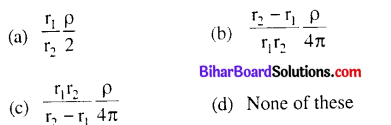(b) $$\frac{r_{2}-r_{1}}{r_{1} r_{2}} \frac{\rho}{4 \pi}$$
Solution: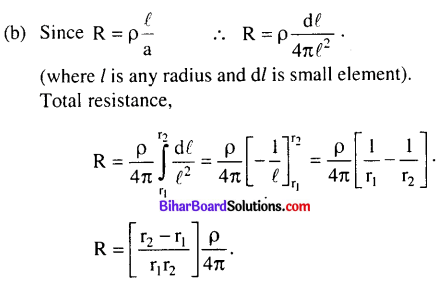Question 7.
The I-V characteristic shown in figure represents
(a) ohmic conductors
(b) non-ohmic conductors
(c) insulators
(d) superconductors
(b) non-ohmic conductorsQuestion 8.
Range of resistivity for metals is
(a) 10-6 Ω m to 10-4 Ω m
(b) 10-7 Ω m to 10-5 Ω m
(c) 10-8 Ω m to 10-6 Ω m
(d) 10-9 Ω m to 10-7 Ω m
(c) 10-8 Ω m to 10-6 Ω m

Question 9.
Wire bound resistors are made by
(a) winding the wires of an alloy viz, Cu, Al, Ag
(b) winding the wires of an alloy viz, Si, Tu, Fe
(c) winding the wires of an alloy viz, Ge, Au, Gr
(d) winding the wires of an alloy viz, manganin, constantan, nichrome.
(d) winding the wires of an alloy viz, manganin, constantan, nichrome.

Question 10.
The resistivity of alloy manganin is
(a) Nearly independent of temperature
(b) Increases rapidly with increase in temperature
(c) Decreases with increase in temperature
(d) Increases rapidly with decrease in temperature
(a) Nearly independent of temperature

Question 11.
What is the order of magnitude of the resistance of a dry human body ?
(a) 10 Ω
(b) 10 KΩ
(c) 10 MΩ
(d) 10 μΩ
(b) 10 KΩ

Question 12.
Two copper wires of length l and 21 have radii, r and 2r respectively. What is the ratio of their specific resistances ?
(a) 1 : 2
(b) 2 : 1
(c) 1 : 1
(d) 1 : 3
(c) 1:1Question 13.
Nichrome or Manganin is widely used in wire bound standard resistors because of their
(a) temperature independent resistivity
(b) very weak temperature dependent resistivity
(c) strong dependence of resistivity with temperature
(d) mechanical strength
(b) very weak temperature dependent resistivity

Question 14.
An electric heater is connected to the voltage supply. After few seconds, current get its steady value then its initial current will be
(a) equal to its steady current
(b) slightly higher than its steady current
(c) slightly less than its steady current
(d) zero
(b) slightly higher than its steady current

Question 15.
In the circuit shown in figure heat developed across 2 Ω,4 Ω and 3 Ωresistances are in the ratio of(a) 2 : 4 : 3
(b) 8 : 4 : 12
(c) 4 : 8 : 27
(d) 8 : 4 : 27
(d) 8 : 4 : 27

Question 16.
The total resistance in the parallel combination of three resistances 9 Ω,7 Ω, and 5Ω is
(a) 1.22 Ω
(b) 2.29 Ω
(c) 4.22 Ω
(d) 2.02 Ω
(d) 2.02 Ω
Solution:
(d) In the parallel combination of three resistances, the equivalent resistance is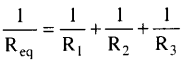Question 17.
Five equal resistances of 10 £2 are connected between A and B as shown in figure. The resultant resistance(a) 10Ω
(b) 5Ω
(c) 15Ω
(d) 6Ω
Answre:
(b) 5Ω
Solution:
(b) According to the given circuit 10 Ω and 10 Ω resistances are connected in series.
∴ R’= 10 + 10 = 20Ω
Again 10 Ω and 10Ω resistances are connected in series
∴R”= 10 + 10 = 20 Ω
R’, R” and 10 Ω all connected in parallel thanQuestion 18.
A copper cylindrical tube has inner radius a and outer radius b. The resistivity is P. The resistance of the cylinder between the two ends is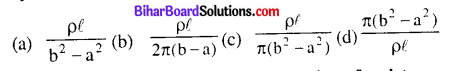(c) $$\frac{\rho^{f}}{\pi\left(b^{2}-a^{2}\right)}$$

Question 19.
A and B are two points on a uniform ring of resistance 15Ω . The ∠AOB = 45°. The equivalent resistance between A and B is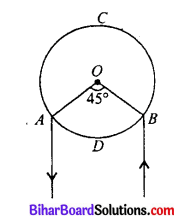(a) 1.64 Ω
(b) 2.84 Ω
(c) 4.57 Ω
(d) 2.64 Ω
(a) 1.64 Ω

Question 20.
The reading of ammeter shown in figure is(a) 6.56 A
(b) 3.28 A
(c) 2.18 A
(d) 1.09 A
(d) 1.09 AQuestion 21.
In a circuit a cell with internal resistance r is connected to an external resistance R. The condition for the maximum current that drawn from the cell is
(a) R = r
(b) R < r
(c) R > r
(d) R = 0
(d) R = 0

Question 22.
In parallel combination of n cells, we obtain
(a) more voltage
(b) more current
(c) less voltage
(d) less current
(b) more current

Question 23.
If n cells each of emf e and internal resistance r are connected in parallel, then the total emf and internal resistances will be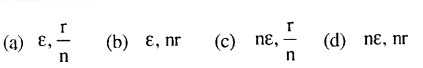(a) $$\varepsilon, \frac{\mathrm{r}}{\mathrm{n}}$$

Question 24.
The potential difference between A and B as shown in figure is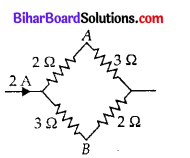(a) 1 V
(b) 2 V
(c) 3 V
(d) 4 V
(a) 1 V
Solution:
(a) Resistance of the upper arm CAD = 2 Ω +3 Ω = 5 Ω
Resistance of the lower arm CBD = 3 Ω +2 Ω =5 Ω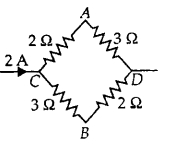As the resistance of both arms are equal, therefore same amount of current flows in both the arms. Current through each arm CAD or CBD = 1APotential difference across C and A is
VC – VB = (2Ω) (1 A)
= 2 V …..(i)
Potential difference across C and B is
VC – VB = (3Ω) (1 A)
= 3 V ………..(ii)
Subtracting (i) from (ii), we get VA – VB = 3V – 2V = 1VQuestion 25.
In a Wheatstone bridge if the battery and galvanometer are interchanged then the deflection in galvanometer will
(a) change in previous direction
(b) not change
(c) change in opposite direction
(d) none of these
(b) not change

Question 26.
In a Wheatstone’s network, P = 2Ω, Q = 2Ω, R = 2Ω, and S = 3Ω The resistance with which S is to be shunted in order that the bridge may be balanced is
(a) 1Ω
(b) 2Ω
(c) 4Ω
(d) 6Ω
(d) 6Ω
Solution:
(d) Let x be the resistance shunted with S for the bridge to be balanced.
For a balance Wheatone’s bridge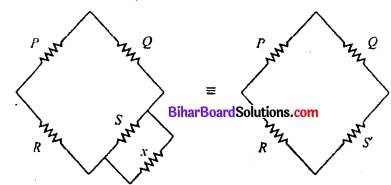Question 27.
When a metal conductor connected to left gap of a meter bridge is heated, the balancing point
(a) shifts towards right
(b) shifts towards left
(c) remains unchanged
(d) remains at zero
(a) shifts towards rightQuestion 28.
In a meter bridge experiment, the ratio of the left gap resistance to right gap resistance is 2 : 3, the balance point from left is
(a) 60 cm
(b) 50 cm
(c) 40 cm
(d) 20 cm
(c) 40 cm
Solution: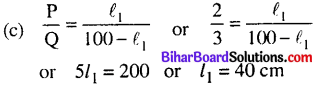Question 29.
Which of the following characteristics of electrons determines the current in a conductor ?
(a) Drift velocity alone
(b) Thermal velocity alone
(c) Both drift velocity and thermal velocity
(d) Neither drift not thermal velocity